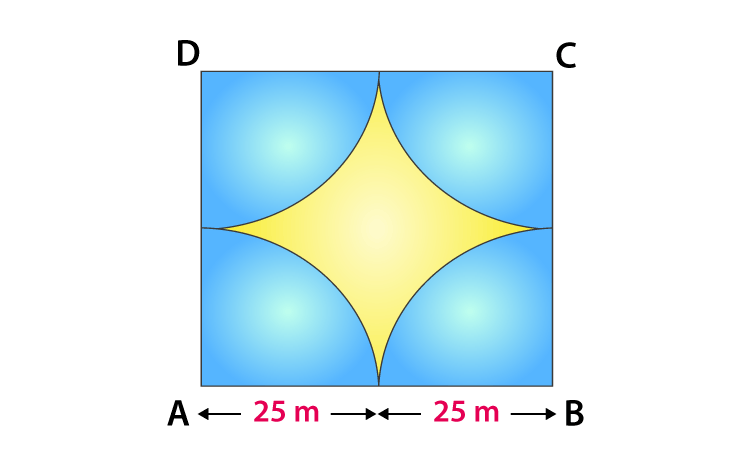# Four cows are tethered at four corners of a square plot of side 50m, so that they just cannot reach one another. What area will be left un-grazed?Given,

Side of square plot = 50 m

Hence, we can say

Area of plot left un-grazed = Area of the plot – 4 x (area of a quadrant)

= Side2 – 4 x {(1/4) x πr2)}

= 502 – (22/7) x (25)2

= 2500 – 1964.28

We get,

= 535.72 m2(0)(0)